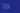# Binary And Data

## Previous Post About How Computers Work

What Makes A Computer A Computer

You may have heard that computers work on 1s and 0s.

Today, almost nobody works with 1s and 0s but they still play a big role in how computers work inside.

Inside a computer are electric wires and circuits that carry all the information in a computer

## How do you store or represent information using electricity

If you have a single wire with electricity flowing through it, the signal can either be on or off. That is not a lot of choices but it is an important start.

With one wire we can represent:

on or off

yes or no

true or false

1 or 0

Or anything else with two options.

This on/off start of a single wire is called a bit. A bit is the smallest piece of information that a computer can store.

If you use more wires, you get more bits. With more bits, you can represent more complex information.

To understand how we can represent complex information with more bits we need to learn about the binary number system.

## The Binary Number System

In the decimal number system, there are 10 digits from zero to nine which is how we all learned to count.

In the binary number system, there are only two digits, 0 and 1. With these two digits, we can count to any number.

### Counting In Binary

Here's how we can use only two digits to count to any number.

In the decimal number system, we are all used to each position in a number that has a different value. These positions start at 1 and multiply by 10 each new position.

With the number 789, 9 is in the one position, 8 is in the ten position, and 7 is in the 100 position.

In the binary number system instead of multiplying each position by 10, we multiply each position by 2.

The starting position would be one, the next is two, then four, eight, and so on.

To calculate the value of the number we are counting to we multiply each digit by the position the digit is in and add all of the results together.

Let's use 1001 as an example.

There are four digits so the positions are: 8, 4, 2, 1.

1 is in the 8 position, 0 is in the 4 position, 0 is in the 2 position, and 1 is in the 1 position.

We know we need to multiply the digit and the position the digit is in which will give us a simple equation:

(1 x 8) + (0 x 4) + (0 x 2) + (1 x 1) = 9

So 1001 in binary would represent the number 9.

### Binary Takeaway

What is important is that any number can be represented with only ones and zeros or by a bunch of wires that are on or off.

The more wires you use, the larger the numbers you can store.

With eight wires, you can store numbers between zero and 255. 255 would be represented by eight ones.

With just 32 wires you can store all the way from 0 to over 4 billion.

Using the binary number system you can represent any number you like, but how do you represent other types of information in binary.

## Information in Binary

How do we represent information like text, images, or sound? It turns out that all of these types of information can also be represented as numbers.

#### Text in Binary

Think of all the letters in the alphabet. We can represent each letter with a number. Those numbers can then be represented in binary using the binary number system defined above.

For example:

A would be the number 1 and represented in binary as 00001

B would be the number 2 and represented in binary as 00010

C would be the number 3 and represented in binary as 00011

We can then represent words and paragraphs as a sequence of numbers.

D-O-G would be 4-15-7 as numbers and 00100-01111-00111 in binary.

And with the binary values, we can store this information as on or off electrical signals.

Every word you see on every webpage or your phone is represented using a system like this.

#### Images in Binary

Now, let's consider photos, videos, and graphics you see on a screen.

All these images are made out of tiny dots called pixels, and each pixel has a color. Each color can be represented as a number which means that each color can be represented in binary.

When you consider that a typical image has millions of pixels and a typical video shows 30 images per second you'll see that we are talking about a lot of data.

#### Sound in Binary

Every sound is basically a series of vibrations in the ear. Vibrations can be represented graphically as a waveform.

Any point on this waveform can be represented by a number.

In this way, any sound can be broken down into a series of numbers.

For higher-quality sound, you would pick 32-bit audio over 8-bit audio. More bits means a higher range of numbers.

## Conclusion

When you use a computer day to day you're not dealing directly with these ones and zeroes but you will be dealing with images, sound, and video.

If you want to understand how computers work on the inside, it all comes down to ones and zeroes and the electrical signals in the circuits behind them.

They are the backbone of how all computers input, store, process, and output information.